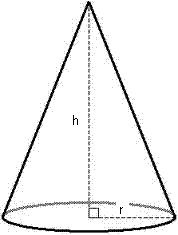# Cone Calculator

Calculate the various properties of a cone for given values.

Slant Height(l) of Cone: [ Sqrt(r² + h²) ]

 Enter the radius : Enter the height : Slant Height(l) of Cone =

Volume of Cone: [ (1/3)πr²h ]

 Enter the radius : Enter the height : Volume of Cone =

Curved Surface Area(CSA) of Cone: [ πrl ]

 Enter the radius : Enter the height : Curved Surface Area(CSA) of Cone =

Total Surface Area(TSA) of Cone: [ πr(l+r) ]

 Enter the radius : Enter the height : Total Surface Area(TSA) of Cone =
A geometrical shape that has a circular base and a single vertex called as Cone. In case vertex is over the center of the base is called a right cone otherwise it is called as oblique cone.
Any object has cone shape is called conical.## Formulas :

Slant Height(l): [ Sqrt(r² + h²) ]

Total Surface Area(TSA): [ πr(l+r) ]

Curved Surface Area(CSA): [ πrl ]

Volume of Cone: [ (1/3)πr²h ]
where:
h = value of height
s = value of slant height
V = value of volume
L = value of lateral surface area
B = value of base surface area
A = value of total surface area
π = pi = value of 3.1415926535898

### Instructions for using surface area of a cone calculator

Understand it step-by-step:

1.For circular base enter the value of the given radius . Depending on the condition different units can be used.

2.Whatever value is known to us based on it you need to enter the height of the cone or the slant height of the cone.

3.Always enter the value of height greater than zero.